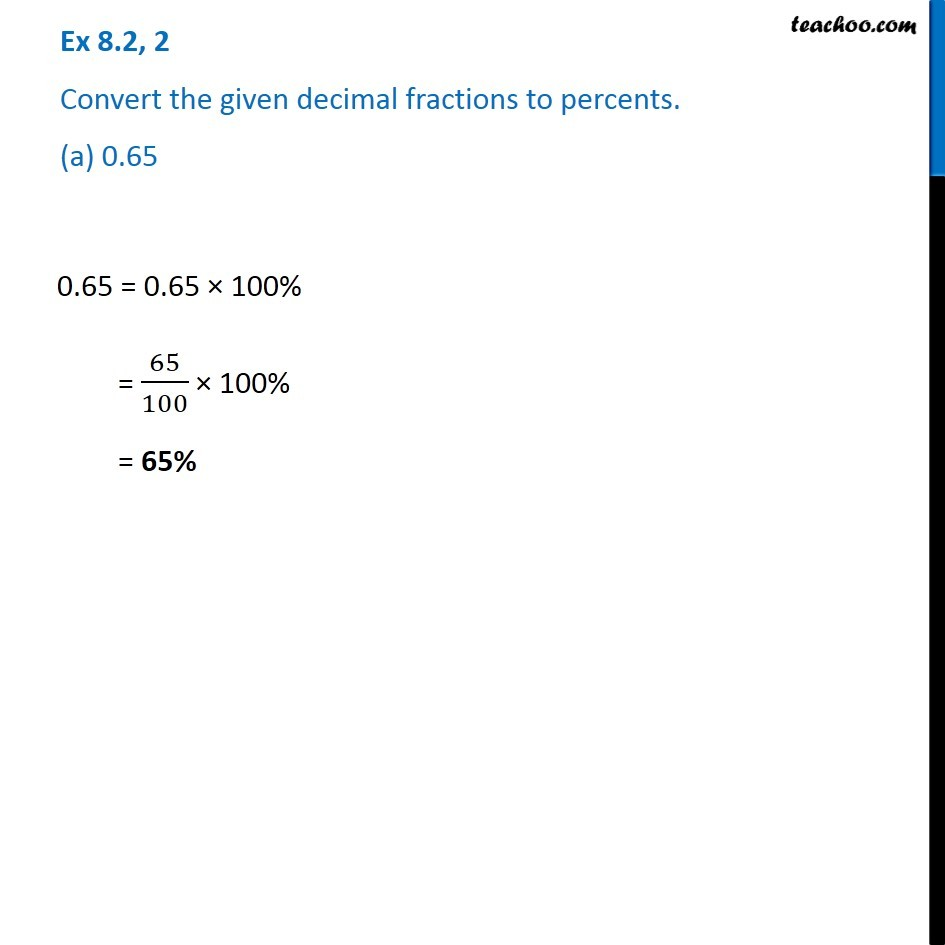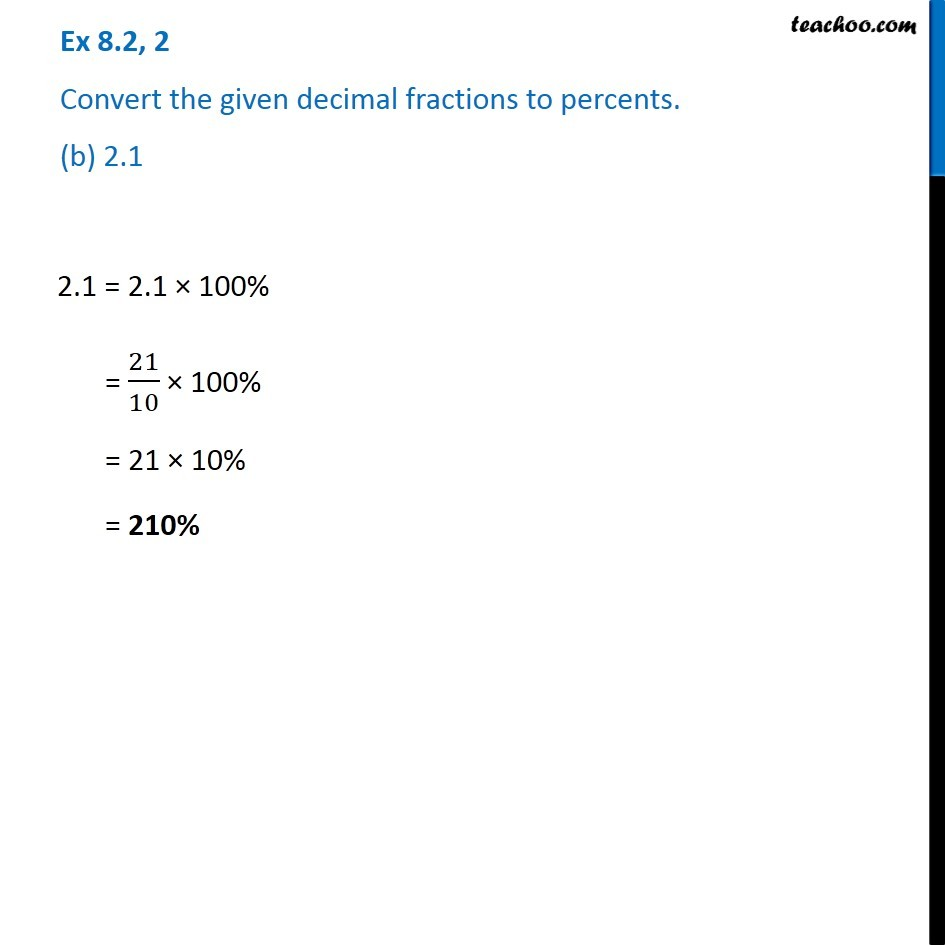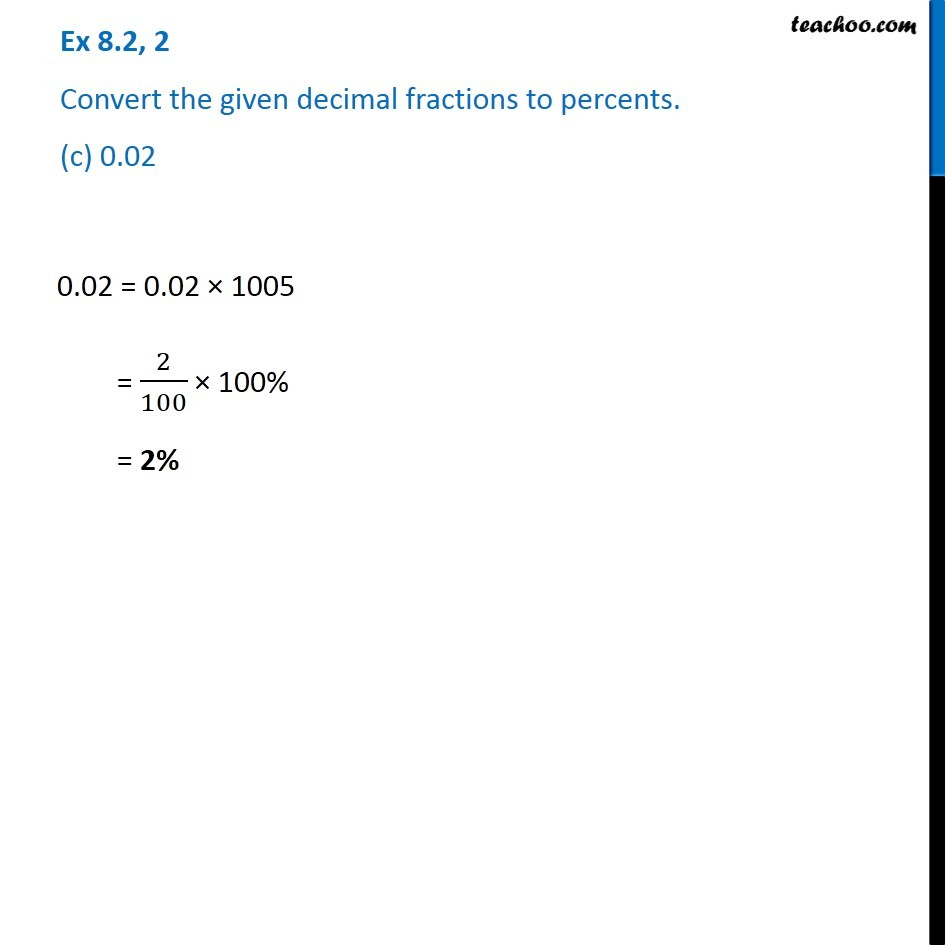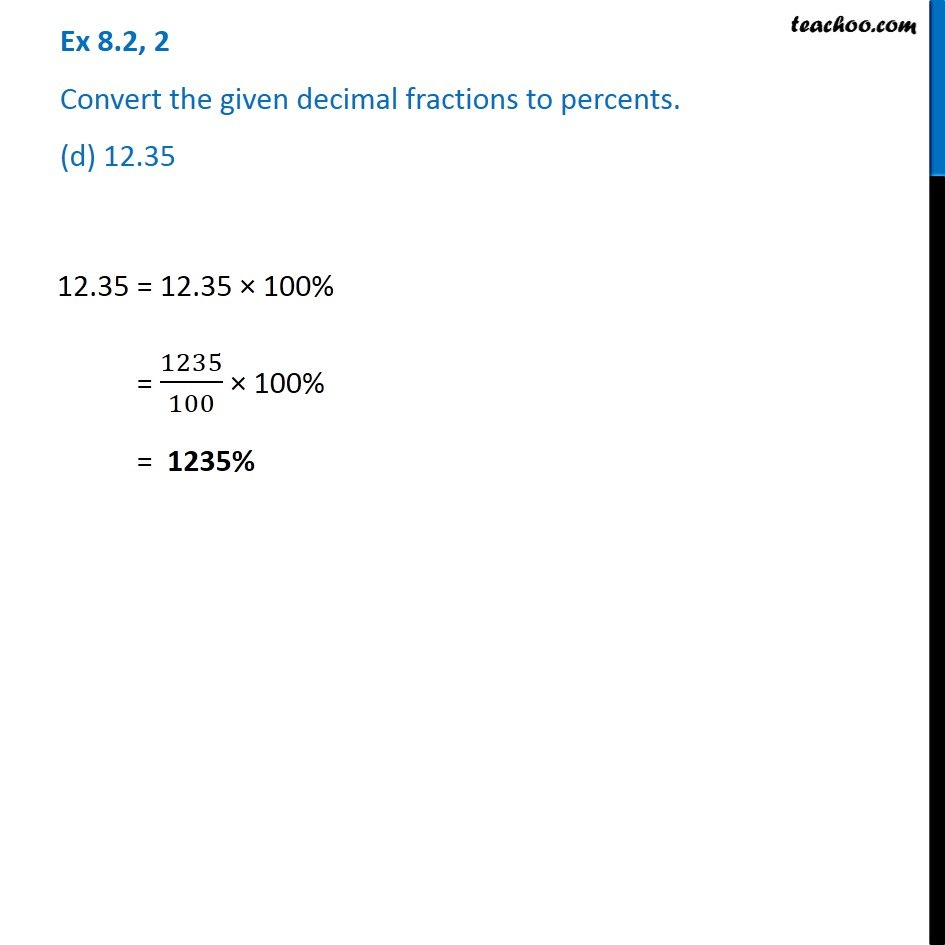1. Chapter 8 Class 7 Comparing Quantities
2. Serial order wise
3. Ex 8.2

Transcript

Ex 8.2, 2 Convert the given decimal fractions to percents. (a) 0.65 0.65 = 0.65 × 100% = 65/100 × 100% = 65% Ex 8.2, 2 Convert the given decimal fractions to percents. (b) 2.1 2.1 = 2.1 × 100% = 21/10 × 100% = 21 × 10% = 210% Ex 8.2, 2 Convert the given decimal fractions to percents. (c) 0.02 0.02 = 0.02 × 1005 = 2/100 × 100% = 2% Ex 8.2, 2 Convert the given decimal fractions to percents. (d) 12.35 12.35 = 12.35 × 100% = 1235/100 × 100% = 1235%

Ex 8.2